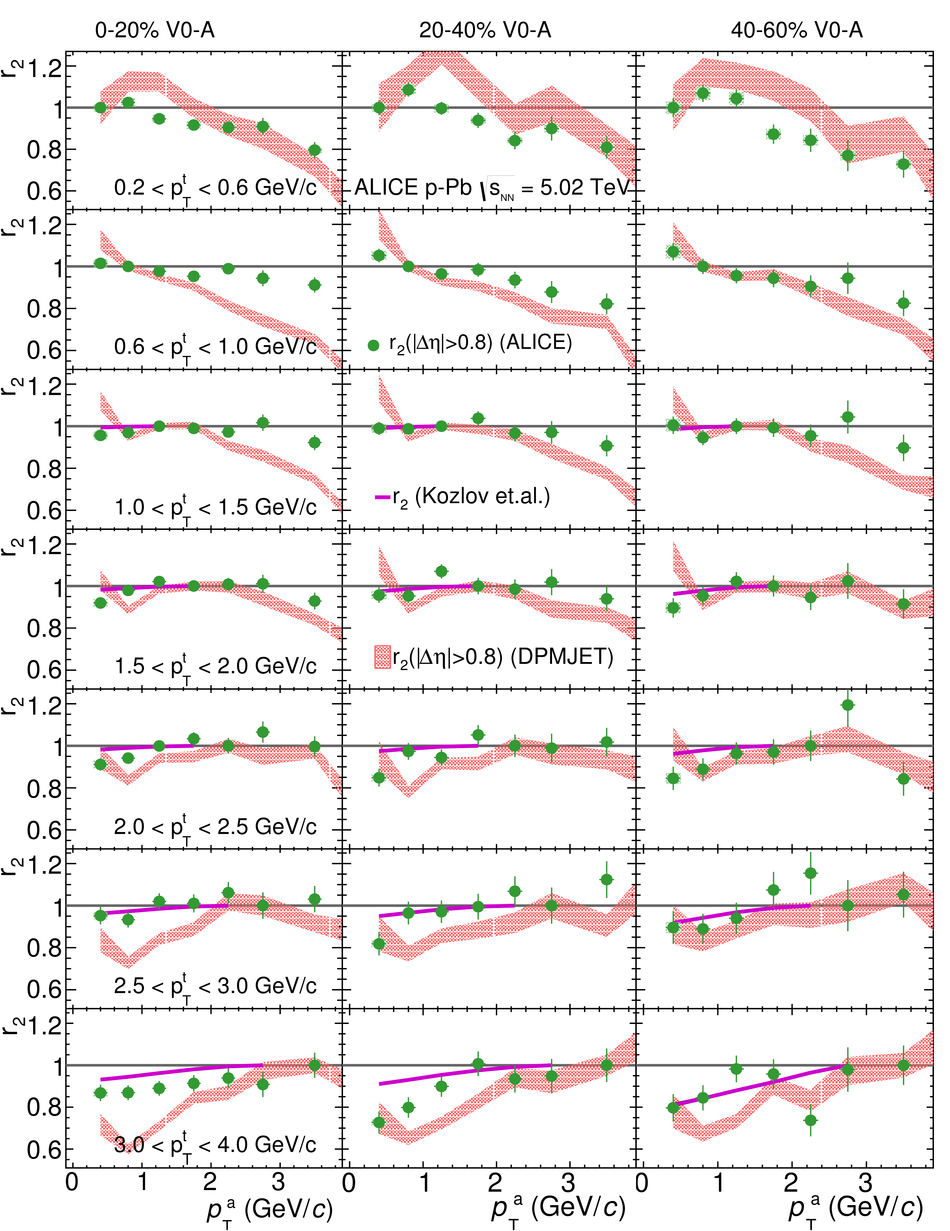# Searches for transverse momentum dependent flow vector fluctuations in Pb-Pb and p-Pb collisions at the LHC

The measurement of azimuthal correlations of charged particles is presented for Pb-Pb collisions at $\sqrt{s_{\rm NN}}=$ 2.76 TeV and p-Pb collisions at $\sqrt{s_{\rm NN}}=$ 5.02 TeV with the ALICE detector at the CERN Large Hadron Collider. These correlations are measured for the second, third and fourth order flow vector in the pseudorapidity region $|\eta|<~0.8$ as a function of centrality and transverse momentum $p_{\rm T}$ using two observables, to search for evidence of $p_{\rm T}$-dependent flow vector fluctuations. For Pb-Pb collisions at 2.76 TeV, the measurements indicate that $p_{\rm T}$-dependent fluctuations are only present for the second order flow vector. Similar results have been found for p-Pb collisions at 5.02 TeV. These measurements are compared to hydrodynamic model calculations with event-by-event geometry fluctuations in the initial state to constrain the initial conditions and transport properties of the matter created in Pb-Pb and p-Pb collisions.

Figures

## Figure 1

 $v_{2}\{2\}$ with $|\Delta\eta|>$ 0 (circles), $|\Delta\eta| >$ 0.4 (diamonds) and $|\Delta\eta| >$ 0.8 (squares) for various centrality classes in Pb--Pb collisions at $\sqrt{s_{_{\rm NN}}} = 2.76$ TeV. Hydrodynamic calculations with MC-Glauber initial conditions and $\eta/s =$ 0.08~, with MC-KLN initial conditions and $\eta/s =$ 0.20~, with Trento initial conditions and temperature dependent $\eta/s$~ and AMPT initial conditions and $\eta/s =$ 0.08~ are shown in green dot-dash, orange dashed curves, and magenta and grey shaded areas, respectively.## Figure 2

 $v_{2}$ with $|\Delta\eta|>$ 0 (circles), $|\Delta\eta| >$ 0.4 (diamonds) and $|\Delta\eta| >$ 0.8 (squares) for various centrality classes in Pb--Pb collisions at $\sqrt{s_{_{\rm NN}}} = 2.76$~TeV. Hydrodynamic calculations with MC-Glauber initial conditions~ and $\eta/s =$ 0.08, with MC-KLN initial conditions and $\eta/s =$ 0.20~, with Trento initial conditions and temperature dependent $\eta/s$~ and AMPT initial conditions and $\eta/s =$ 0.08~ are shown in green dot-dashed and orange dashed curves, and magenta and grey shaded areas, respectively.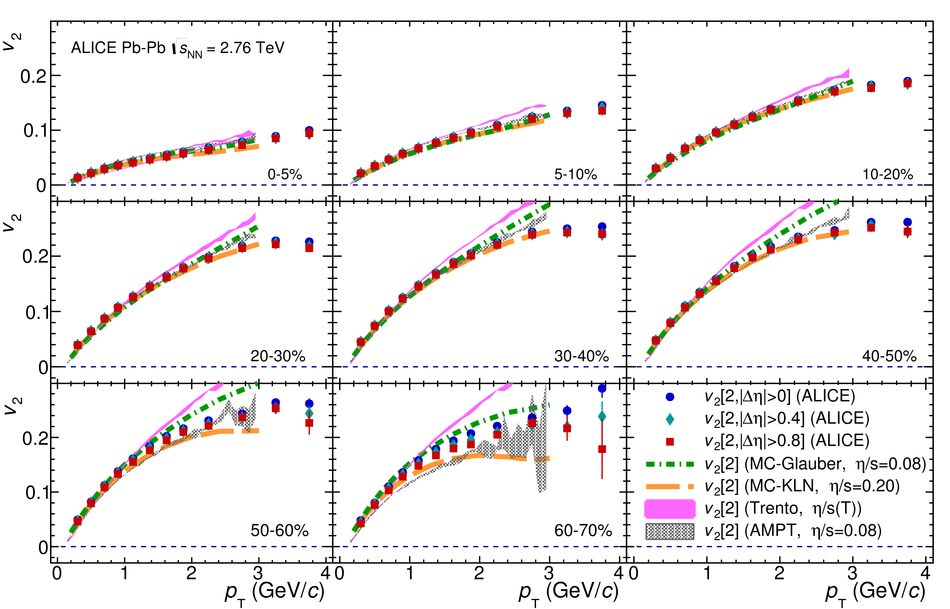## Figure 3

 The ratio $v_{2}\{2, |\Delta\eta| > 0.8\} / v_{2}[2, |\Delta\eta| > 0.8]$ in Pb--Pb collisions at $\sqrt{s_{_{\rm NN}}} = 2.76$~TeV. The different panels show the centrality evolution of the measurements Hydrodynamic calculations with MC-Glauber initial conditions and $\eta/s =$ 0.08~, with MC-KLN initial conditions and $\eta/s =$ 0.20~, with Trento initial conditions and temperature dependent $\eta/s$~ and AMPT initial conditions and $\eta/s =$ 0.08~ are shown in green dot-dashed and orange dashed curves, and magenta and grey shaded areas, respectively.## Figure 4

 $v_{3}\{2\}$ with different $|\Delta\eta|$ gaps is presented in Pb--Pb collisions at $\sqrt{s_{_{\rm NN}}} = 2.76$~TeV. $v_{3}\{2, |\Delta\eta|> 0\}$, $v_{3}\{2, |\Delta\eta|> 0.4\}$, and $v_{3}\{2, |\Delta\eta|> 0.8\}$ are represented by circles, diamonds and squares, respectively. The different panels show the centrality evolution of the measurements Hydrodynamic calculations with MC-Glauber initial conditions and $\eta/s =$ 0.08~, with MC-KLN initial conditions and $\eta/s =$ 0.20~, with Trento initial conditions and temperature dependent $\eta/s$~ and AMPT initial conditions and $\eta/s =$ 0.08~ are shown in green dot-dash, orange dashed curves, and magenta and grey shaded areas, respectively.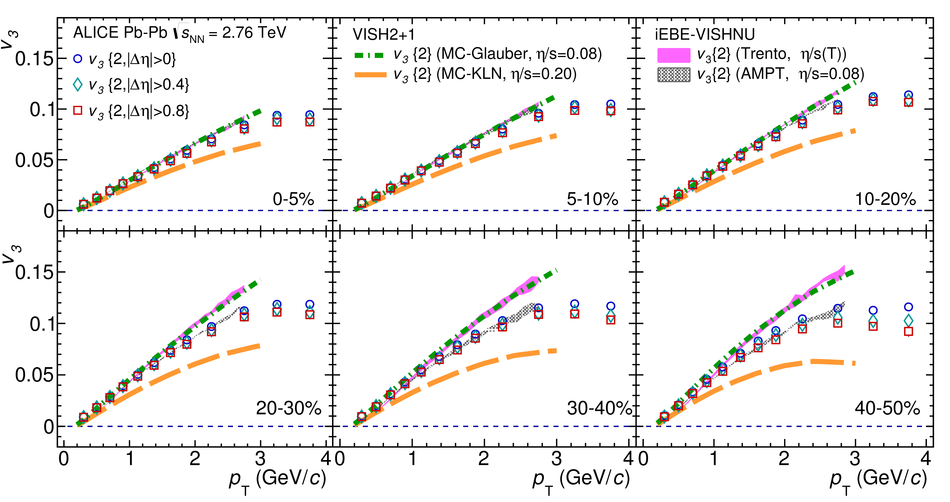## Figure 5

 $v_{3}$ with different $|\Delta\eta|$ gaps is presented in Pb--Pb collisions at $\sqrt{s_{_{\rm NN}}} = 2.76$~TeV. $v_{3}[2, |\Delta\eta|> 0]$, $v_{3}[2, |\Delta\eta|> 0.4]$, and $v_{3}[2, |\Delta\eta|> 0.8]$ are represented by circles, diamonds, and squares, respectively. The different panels show the centrality evolution of the measurements Hydrodynamic calculations with MC-Glauber initial conditions and $\eta/s =$ 0.08~ and with MC-KLN initial conditions and $\eta/s =$ 0.20~, with Trento initial conditions and temperature dependent $\eta/s$~ and AMPT initial conditions and $\eta/s =$ 0.08~ are shown in green dot-dash, orange dashed curves, and magenta and grey shaded areas, respectively.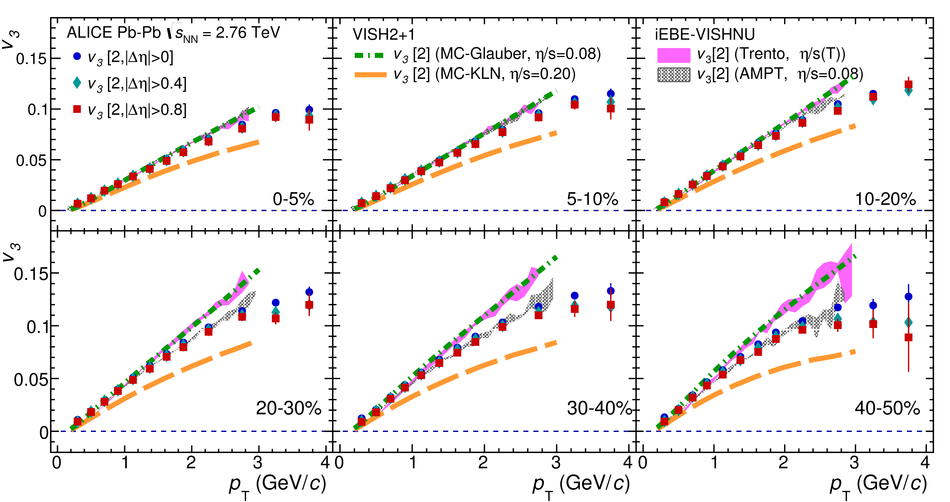## Figure 6

 The ratio $v_{3}\{2, |\Delta\eta| > 0.8\} / v_{3}[2, |\Delta\eta| > 0.8]$ in Pb--Pb collisions at $\sqrt{s_{_{\rm NN}}} = 2.76$~TeV. The different panels show the centrality evolution of the measurements Hydrodynamic calculations with MC-Glauber initial conditions and $\eta/s =$ 0.08~ and with MC-KLN initial conditions and $\eta/s =$ 0.20~, with Trento initial conditions and temperature dependent $\eta/s$~ and AMPT initial conditions and $\eta/s =$ 0.08~ are shown in green dot-dash, orange dashed curves, and magenta and grey shaded areas, respectively.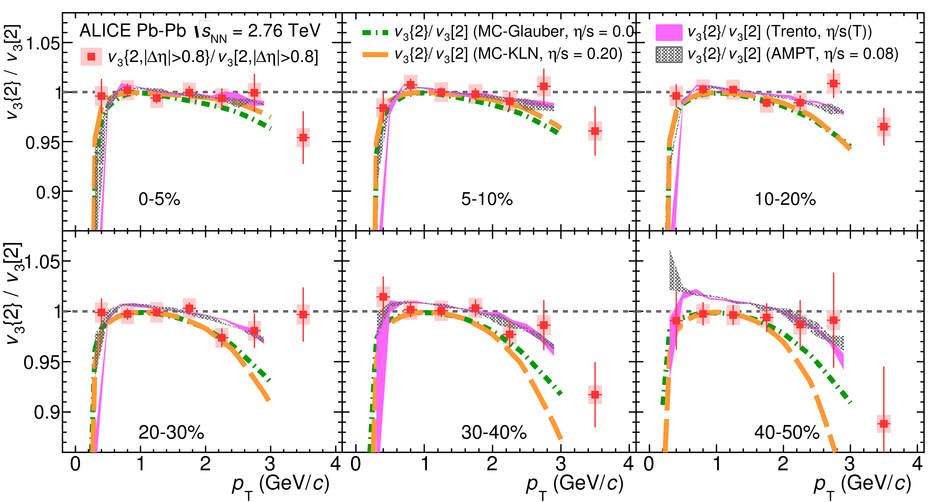## Figure 7

 $v_{4}\{2\}$ with different $|\Delta\eta|$ gaps is presented in Pb--Pb collisions at $\sqrt{s_{_{\rm NN}}} = 2.76$~TeV. $v_{4}\{2, |\Delta\eta|> 0\}$, $v_{4}\{2, |\Delta\eta|> 0.4\}$ and $v_{4}\{2, |\Delta\eta|> 0.8\}$ are represented by circles, diamonds, and squares, respectively. The different panels show the centrality evolution of the measurements Hydrodynamic calculations with MC-Glauber initial conditions and $\eta/s =$ 0.08~, with MC-KLN initial conditions and $\eta/s =$ 0.20~, with Trento initial conditions and temperature dependent $\eta/s$~ and AMPT initial conditions and $\eta/s =$ 0.08~ are shown in green dot-dash, orange dashed curves, and magenta and grey shaded areas, respectively.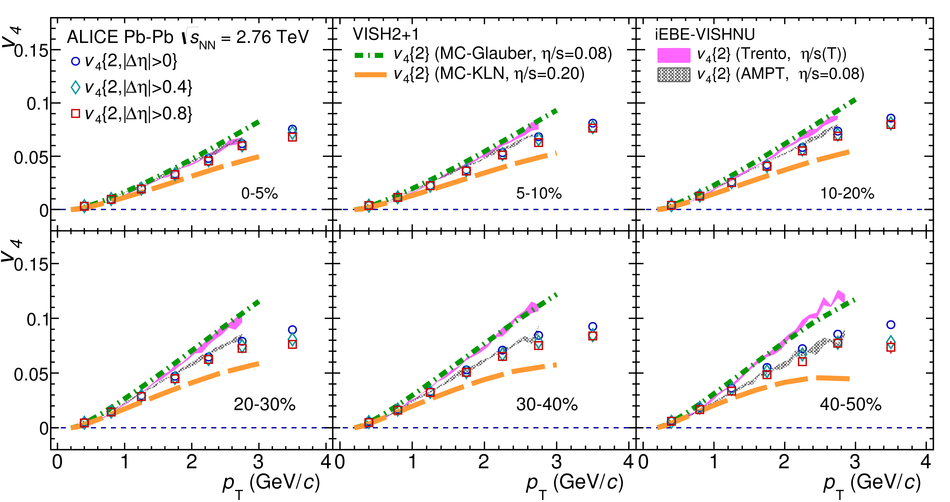## Figure 8

 $v_{4}$ with different $|\Delta\eta|$ gaps is presented in Pb--Pb collisions at $\sqrt{s_{_{\rm NN}}} = 2.76$~TeV. $v_{4}[2, |\Delta\eta|> 0]$, $v_{4}[2, |\Delta\eta|> 0.4]$, and $v_{4}[2, |\Delta\eta|> 0.8]$ are represented by circles, diamonds, and squares, respectively. The different panels show the centrality evolution of the measurements Hydrodynamic calculations with MC-Glauber initial conditions and $\eta/s =$ 0.08~ and with MC-KLN initial conditions and $\eta/s =$ 0.20~, with Trento initial conditions and temperature dependent $\eta/s$~ and AMPT initial conditions and $\eta/s =$ 0.08~ are shown in green dot-dash, orange dashed curves, and magenta and grey shaded areas, respectively.## Figure 9

 The ratio $v_{4}\{2, |\Delta\eta| > 0.8\} / v_{4}[2, |\Delta\eta| > 0.8]$ in Pb--Pb collisions at $\sqrt{s_{_{\rm NN}}} = 2.76$~TeV. The different panels show the centrality evolution of the measurements. Hydrodynamic calculations with MC-Glauber initial conditions and $\eta/s =$ 0.08~ and with MC-KLN initial conditions and $\eta/s =$ 0.20~, with Trento initial conditions and temperature dependent $\eta/s$~ and AMPT initial conditions and $\eta/s =$ 0.08~ are shown in green dot-dash, orange dashed curves, and magenta and grey shaded areas, respectively.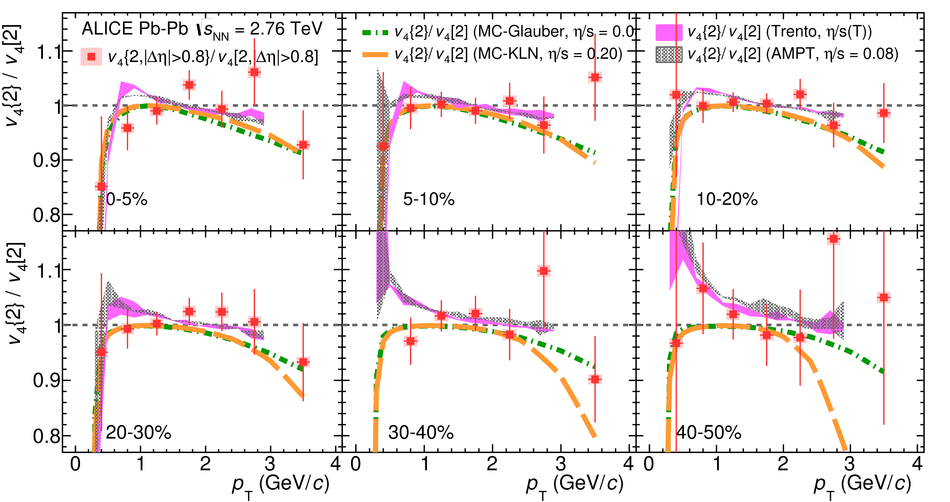## Figure 10

 The factorisation ratio $r_{2}$, as a function of $p_{\rm T}^{\, a}$ in bins of $p_{\rm T}^{t}$ for 0--5$\%$, 20--30$\%$ and 40--50$\%$ centralities in Pb--Pb collisions at $\sqrt{s_{_{\rm NN}}} = 2.76$~TeV, is presented (solid circles). CMS measurements are presented by open square~. Hydrodynamic calculations with MC-Glauber initial conditions and $\eta/s =$ 0.08~ and with MC-KLN initial conditions and $\eta/s =$ 0.20~, with Trento initial conditions and temperature dependent $\eta/s$~ and AMPT initial conditions and $\eta/s =$ 0.08~ are shown in green dot-dash, orange dashed curves, and magenta and grey shaded areas, respectively.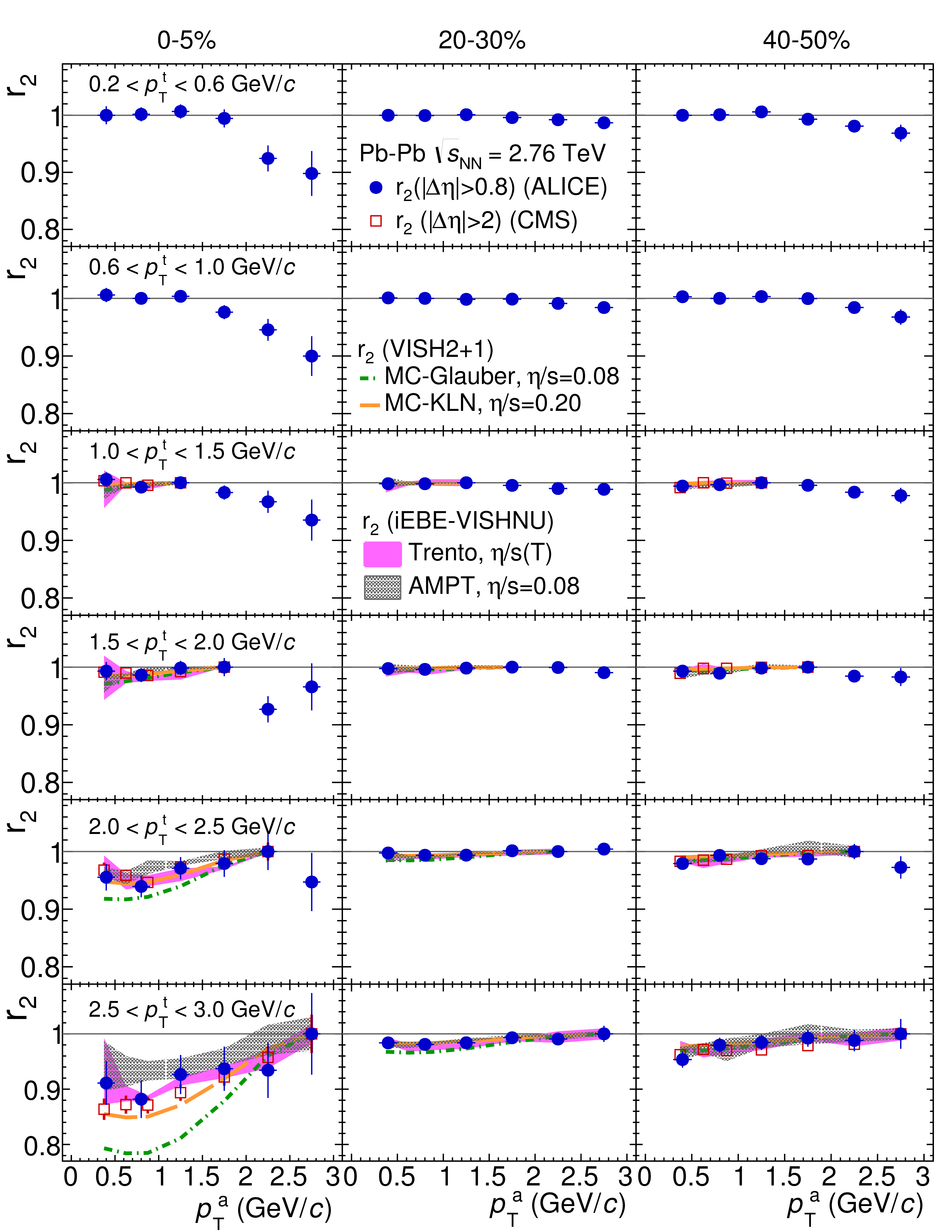## Figure 11

 The factorisation ratio $r_{3}$, as a function of $p_{\rm T}^{\, a}$ in bins of $p_{\rm T}^{t}$ for 0--5$\%$, 20--30$\%$ and 40--50$\%$ centralities in Pb--Pb collisions at $\sqrt{s_{_{\rm NN}}} = 2.76$~TeV, is presented (solid circles). CMS measurements~ are presented by open squares. Hydrodynamic calculations with MC-Glauber initial conditions and $\eta/s =$ 0.08~ and with MC-KLN initial conditions and $\eta/s =$ 0.20~, with Trento initial conditions and temperature dependent $\eta/s$~ and AMPT initial conditions and $\eta/s =$ 0.08~ are shown in green dot-dash, orange dashed curves, and magenta and grey shaded areas, respectively.## Figure 12

 $v_{2}\{2, |\Delta\eta| > 0\}$, $v_{2}[2, |\Delta\eta| > 0]$, $v_{2}\{2, |\Delta\eta| > 0.8\}$ and $v_{2}[2, |\Delta\eta| > 0.8]$ for various multiplicity classes of p--Pb collisions at $\sqrt{s_{_{\rm NN}}} = 5.02$~TeV. DPMJET calculations are presented by red shaded lines for $v_{2}\{2, |\Delta\eta| > 0.8\}$ and blue shaded lines for $v_{2}[2, |\Delta\eta| > 0.8]$ Hydrodynamic calculations (MUSIC) with modified MC-Glauber initial conditions and $\eta/s =$ 0.08 for $v_{2}\{2\}$ and $v_{2}$ are shown in solid blue and dashed red lines.~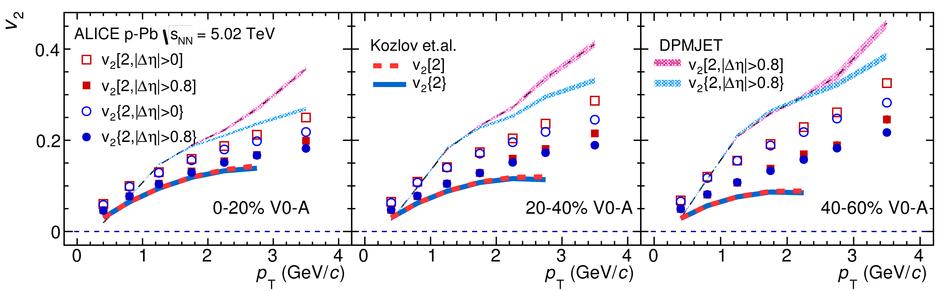## Figure 13

 The ratio $v_{2}\{2, |\Delta\eta| > 0.8\} / v_{2}[2, |\Delta\eta| > 0.8]$ for various multiplicity classes in p--Pb collisions at $\sqrt{s_{_{\rm NN}}} = 5.02$~TeV. DPMJET calculations are presented by green shaded lines Hydrodynamic calculations (MUSIC)~ with modified MC-Glauber initial conditions and $\eta/s =$ 0.08 are shown as solid blue lines.## Figure 14

 $v_{3}\{2, |\Delta\eta| > 0\}$, $v_{3}[2, |\Delta\eta| > 0]$, $v_{3}\{2, |\Delta\eta| > 0.8\}$ and $v_{3}[2, |\Delta\eta| > 0.8]$ for various multiplicity classes in p--Pb collisions at $\sqrt{s_{_{\rm NN}}} = 5.02$~TeV. Hydrodynamic calculations (MUSIC)~ with modified MC-Glauber initial conditions and $\eta/s =$ 0.08 for $v_{2}\{2\}$ and $v_{2}$ are shown as solid blue and dashed red lines.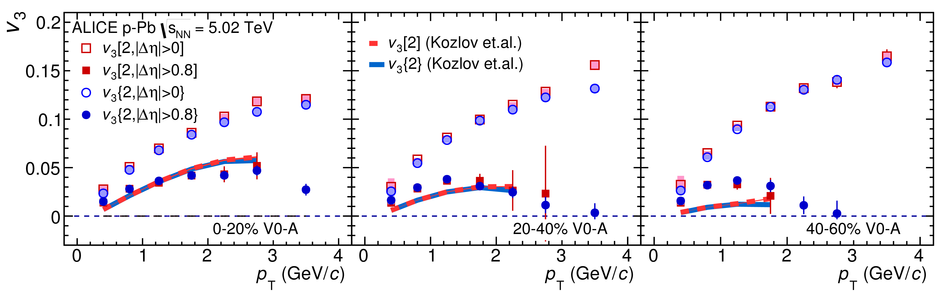## Figure 15

 The factorisation ratio $r_{2}$, as a function of $p_{\rm T}^{\, a}$ in bins of $p_{\rm T}^{t}$ for multiplicity classes 0--20$\%$, 20--40$\%$ and 40--60$\%$ in p--Pb collisions at $\sqrt{s_{_{\rm NN}}} = 5.02$~TeV, are presented by solid magenta circles DPMJET calculations are presented by pink shaded areas Hydrodynamic calculations (MUSIC) with modified MC-Glauber initial conditions and $\eta/s =$ 0.08 are shown as magenta lines~.